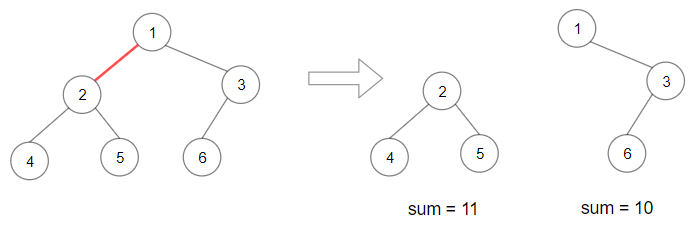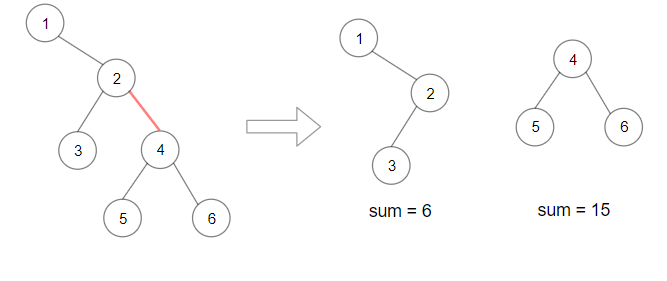## 1339. Maximum Product of Splitted Binary Tree

Given a binary tree `root`. Split the binary tree into two subtrees by removing 1 edge such that the product of the sums of the subtrees are maximized.

Since the answer may be too large, return it modulo 10^9 + 7.

Example 1:```Input: root = [1,2,3,4,5,6]
Output: 110
Explanation: Remove the red edge and get 2 binary trees with sum 11 and 10. Their product is 110 (11*10)
```

Example 2:```Input: root = [1,null,2,3,4,null,null,5,6]
Output: 90
Explanation:  Remove the red edge and get 2 binary trees with sum 15 and 6.Their product is 90 (15*6)
```

Example 3:

```Input: root = [2,3,9,10,7,8,6,5,4,11,1]
Output: 1025
```

Example 4:

```Input: root = [1,1]
Output: 1
```

Constraints:

• Each tree has at most `50000` nodes and at least `2` nodes.
• Each node's value is between `[1, 10000]`.

## Rust Solution

``````struct Solution;
use rustgym_util::*;

trait Postorder {
fn sum(&self) -> i32;
fn product(&self, max: &mut i64, sum: i32) -> i32;
}

fn sum(&self) -> i32 {
if let Some(node) = self {
let node = node.borrow();
let val = node.val;
let left = node.left.sum();
let right = node.right.sum();
val + left + right
} else {
0
}
}

fn product(&self, max: &mut i64, sum: i32) -> i32 {
if let Some(node) = self {
let node = node.borrow();
let val = node.val;
let left = node.left.product(max, sum);
let right = node.right.product(max, sum);
let res = val + left + right;
*max = (*max).max((sum - res) as i64 * res as i64);
res
} else {
0
}
}
}

impl Solution {
fn max_product(root: TreeLink) -> i32 {
let sum = root.sum();
let mut res = 0;
root.product(&mut res, sum);
(res % 1_000_000_007) as i32
}
}

#[test]
fn test() {
let root = tree!(1, tree!(2, tree!(4), tree!(5)), tree!(3, tree!(6), None));
let res = 110;
assert_eq!(Solution::max_product(root), res);
let root = tree!(1, None, tree!(2, tree!(3), tree!(4, tree!(5), tree!(6))));
let res = 90;
assert_eq!(Solution::max_product(root), res);
let root = tree!(1, tree!(1), None);
let res = 1;
assert_eq!(Solution::max_product(root), res);
}
``````

Having problems with this solution? Click here to submit an issue on github.